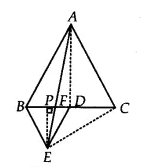Q

# In Fig.9.33, ABC and BDE are two equilateral triangles such that D is the mid-point of BC. If AE intersects BC at F, show that (ii) ar (BDE) = 1/2 ar (BAE)

Q: 5    In Fig. , ABC and BDE are two equilateral triangles such that D is the mid-point of BC. If AE intersects BC at F, show that

(ii)

[Hint: Join EC and AD. Show that and  , etc.]ViewsSince ABC and  BDE are equilateral triangles.
Therefore, ACB = DBE =
BE || AC

BAE and BEC are on the same base BE and between same parallels BE and AC.
Therefore, ar (BAE) = ar(BEC)
ar(BAE) = 2 ar(BED)   [since D is the meian of BEC ]

Hence proved.

Exams
Articles
Questions